Next: Mesh Size Specification Up: T3d User Guide Previous: Contents

# Model Representation

The model is described by a boundary representation and consists of the following model entities:

• vertices,
• curves,
• surfaces,
• patches,
• shells, and
• regions.
Topologically, each region is formed by a set of not self-intersecting boundary surfaces, patches, and shells, each of which is bounded by a set of curves. Each curve is given by two end vertices. Moreover, each boundary surface, patch, and shell points out to the regions on the side of its outer and inner normal. A curve keeps list of surfaces, patches, and shells sharing that curve. Similarly, a vertex stores the list of curves sharing that vertex. This basic topology is further restricted by the geometry of model entities. Both curves and surfaces are based on free-form representation in terms of tensor-product polynomial entities. This limits the number of curves bounding a surface to four. The number of curves bounding a patch or a shell is not limited (but must be at least two). While the patch is a planar model entity (trimmed plane), shell is constrained to a background surface (trimmed surface). To enhance the modelling capability an entity-to-entity fixation concept has been introduced. Generally, each model entity may be fixed to another model entity of the same or higher dimension. However, the fixed entity is not allowed to coincide with the boundary of the parent entity. Each model entity keeps the list of entities fixed to it. No further topological information is required for the description of a valid non-manifold domain of almost arbitrary complexity.

Currently, rational Bezier entities are employed for free-form curves and surfaces representation. This allows to represent exactly conics and quadrics by entities starting with an order of three (quadratic curves and biquadratic surfaces).

The rational Bezier curve has the form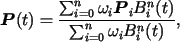(1)

where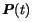is the point on the curve,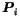are Bezier control points,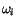are weights of Bezier control points,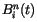stand for Bernstein polynomials,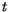denotes an independent variable varying in range from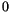to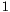, and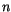is the curve degree. The curve order is equal to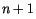.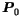and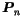correspond to model vertices while the remaining points form the control polygon of the curve. They determine the bow of the curve and need not generally lie on the curve. The first and last segments of the control polygon coincide with the curve tangent in the starting and ending vertices respectively.

The rational Bezier surface can be written in a similar form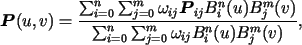(2)

where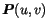is the point on the surface,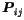are Bezier control points,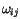are weights of Bezier control points,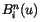and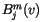stand for Bernstein polynomials,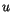and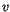denote independent parameters varying in range fromto, andand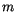are surface degrees (orders are equal toand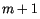) inandparametric directions, respectively. If the control points are arranged in a matrix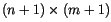then the corner points correspond to model vertices, the side points correspond to control polygons of model curves bounding the surface, and the remaining points form the control polygon of the surface and need not generally lie on the surface.

Bernstein polynomial can be expressed as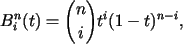(3)

or recursively as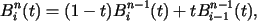(4)

where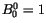.

The ordinary Bezier entities can be derived from rational Bezier entities when all weights are set to 1.

Two types of model entities are distinguished. The physical ones which are designated for the actual discretization and the virtual ones which serve as auxiliary for geometry description or mesh size specification. Note that there are some restrictions on the fixation between virtual and physical entities.Next: Mesh Size Specification Up: T3d User Guide Previous: Contents

Daniel Rypl
2008-06-04